Transforming Variables

# Other Transformations

You can use the Edit Variables dialog to create other types of transformations. Most transformations require one selected variable, as in the previous example. Here is an example using two variables. Suppose you are interested in batting averages, that is, the number of hits per batting opportunity. Calculate batting averages by following these steps.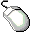Choose Edit:Variables:Other to display the Edit Variables dialogAssign NO_HITS the Y role and NO_ATBAT the X role.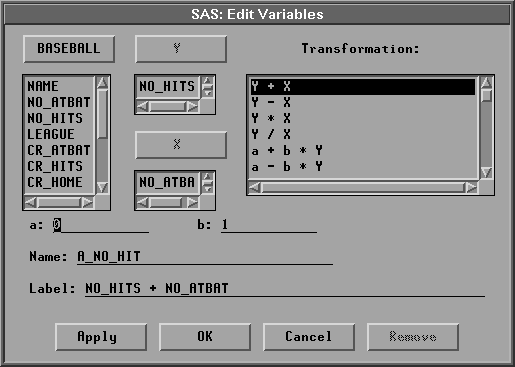Figure 20.14: Edit Variables DialogClick on the Y / X transformation.

Notice that the Label value is now NO_HITS / NO_ATBAT. You might want to enter a more mnemonic value for Name.Enter BAT_AVG in the Name field.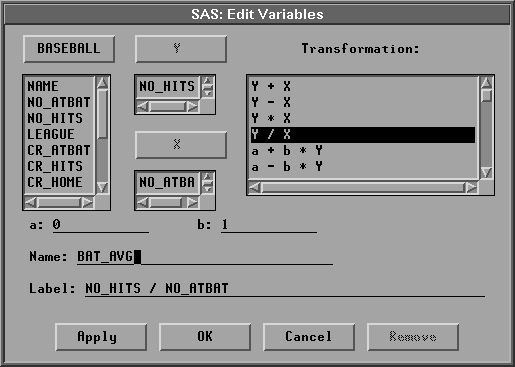Figure 20.15: Creating the TransformationClick the OK button to calculate the batting average.

The new BAT_AVG variable appears at the last position in the data window.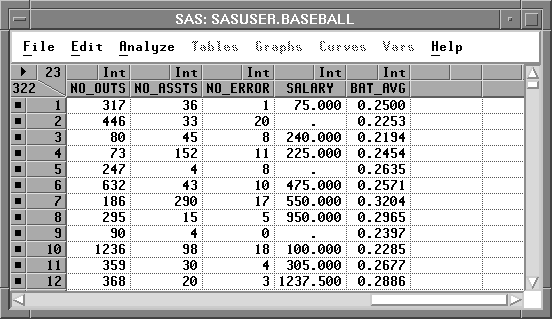Figure 20.16: New BAT_AVG Variable

Now look at the distribution of batting averages for each league by creating a box plot.Choose Analyze:Box Plot/Mosaic Plot ( Y ).

Specify BAT_AVG as the Y variable, LEAGUE as the X variable, and NAME for the Label role in the box plot variables dialog. Then click on OK.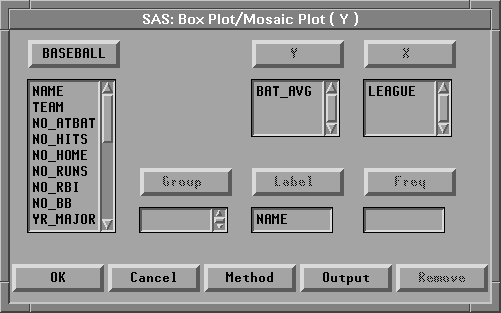Figure 20.17: Box Plot Dialog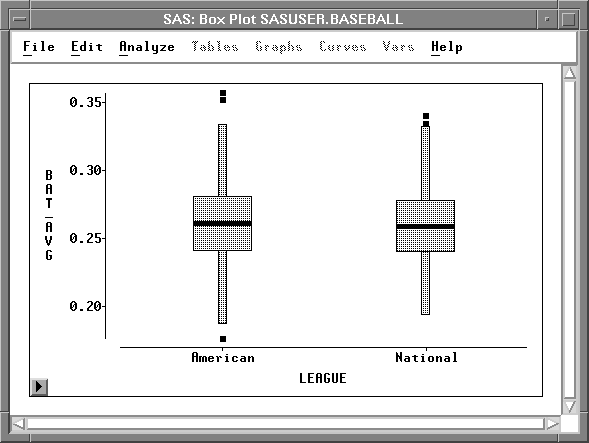Figure 20.18: Box Plot of Batting Averages

Most players are batting between .200 and .300. There are, however, a few extreme observations.Select the upper extreme observations for each league.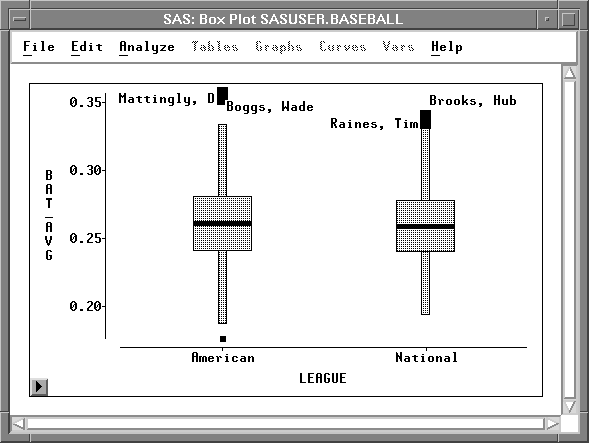Figure 20.19: Examining the Extreme Observations

Don Mattingly and Wade Boggs led the American League in batting, while Tim Raines and Hubie Brooks led the National League.

The Edit:Variables menu and dialog offer many other transformations. Here is the complete list of transformations in the Edit:Variables menu:

log( Y )
calculates the natural logarithm of the Y variable.

sqrt( Y )
calculates the square root of the Y variable.

1 / Y
calculates the reciprocal of the Y variable.

Y * Y
calculates the square of the Y variable.

exp( Y )
raises e (2.718...) to the power given by the Y variable.

Here is the complete list of transformations in the Edit:Variables dialog:

 Y + X Y - X Y * X Y / X These four transformations perform addition, subtraction, multiplication, and division on the specified Y and X variables. a + b * Y a     - b * Y a + b / Y a     - b / Y These four transformations create linear transformations of the Y variable. Using the default values a=0 and b=1, the second and third transformations create additive and multiplicative inverses -Y and 1 / Y. Y ** b is the power transform. b can be positive or negative. (( Y + a ) ** b - 1 ) / b is the Box-Cox transformation. This transformation raises the sum of the Y variable plus a to the power b, then subtracts 1 and divides by b. a <= Y <= b creates a variable with value 1 when the value of Y is between a and b inclusively, and value 0 for all other values of Y. Values for a and b can be character or numeric; character values should not be in quotations. You can use this transformation to create indicator variables for subsetting your data. (Y - mean(Y)) / std(Y) standardizes the Y variable by subtracting its mean and dividing by its standard deviation. Standardizing changes the mean of the variable to 0 and its standard deviation to 1. abs( Y ) calculates the absolute value of Y. arccos( Y ) calculates the arccosine (inverse cosine) of Y. The value is returned in radians. arcsin( Y ) calculates the arcsine (inverse sine) of Y. The value is returned in radians. arcsin( sqrt( Y )) calculates the arcsine of the square root of Y. The value is returned in radians. arctan( Y ) calculates the arctangent (inverse tangent) of Y. The value is returned in radians. ceil( Y ) calculates the smallest integer greater than or equal to Y. cos( Y ) calculates the cosine of Y. exp( Y ) raises e (2.718...) to the power given by the Y variable. floor( Y ) calculates the largest integer less than or equal to Y. log( Y + a ) calculates the natural logarithm of the Y variable plus an offset a. log2( Y + a ) calculates the logarithm base 2 of the Y variable plus an offset a. log10( Y + a ) calculates the logarithm base 10 of the Y variable plus an offset a. log(( Y - a ) / ( b - Y )) calculates the natural logarithm of the quotient of the Y variable minus a divided by b minus the Y variable. When a = 0 and b = 1, this is a logit transformation. ranbin( a, b ) generates a binomial random variable containing values either 0 or 1. a is the seed value for the random transformation. b is the probability that the generated value will be 1. If a is less than or equal to 0, the time of day is used. This is a special case of the SAS function RANBIN where n, the number of trials, is 1. ranexp( a ) generates a random variable from an exponential distribution. a is the seed value for the random transformation. If a is less than or equal to 0, the time of day is used. rangam( a, b ) generates a random variable from a gamma distribution. a is the seed value for the random transformation, and b is the shape parameter. If a is less than or equal to 0, the time of day is used. rannor( a ) generates a random variable from a normal distribution with mean 0 and variance 1. a is the seed value for the random transformation. If a is less than or equal to 0, the time of day is used. ranpoi( a, b ) generates a random variable from a Poisson distribution. a is the seed value for the random transformation, and b is the mean parameter. If a is less than or equal to 0, the time of day is used. ranuni( a ) generates a uniform random variable containing values between 0 and 1. a is the seed value for the random transformation. If a is less than or equal to 0, the time of day is used. round( Y ) calculates the nearest integer to Y. sin( Y ) calculates the sine of Y. sqrt( Y + a ) calculates the square root of the Y variable plus an offset a. tan( Y ) calculates the tangent of Y.

If your work requires other transformations that do not appear in the Edit:Variables menu or in the Edit Variables dialog, you can perform many kinds of transformations using the SAS DATA step. For more complete descriptions of the ranbin, ranexp, rangam, rannor, ranpoi, and ranuni transformations and for complete information on the DATA step, refer to SAS Language Reference: Dictionary.